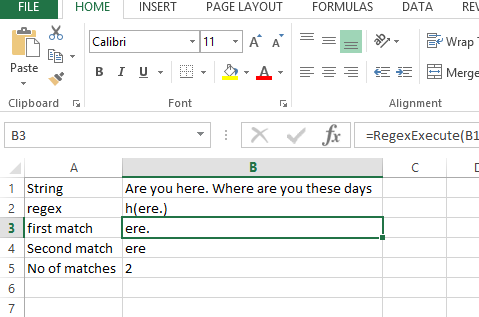### Regular Expressions (Regex) in Excel VBA

In this article we will learn to use the Regex in Excel:

Excel does not natively provide any Regex functions which often requires creating complex formulas for extracting pieces of strings otherwise easy to extract using Regular Expressions. Hence, to facilitate Regex in Excel you need to use User Defined Functions – functions defined in VBA but accessible as regular functions in Excel. Below find 2 basic UDF functions created just for this use:

Let us write the following function in Excel and save the file as macro enabled file:You can copy from here as well or you can check the file attached at the end:

```'Returns the number of matches found for a given regex
'str - string to test the regex on
'reg - the regular expression
Public Function RegexCountMatches(str As String,
reg As String) As String
On Error GoTo ErrHandl
Set regex = CreateObject("VBScript.RegExp"): regex.Pattern
= reg: regex.Global = True
If regex.test(str) Then
Set matches = regex.Execute(str)
RegexCountMatches = matches.Count
Exit Function
End If
ErrHandl:
RegexCountMatches = CVErr(xlErrValue)
End Function

'Executes a Regular Expression on a provided string and
returns a selected submatch
'str - string to execute the regex on
'reg - the regular expression with at least 1 capture '()'
'matchIndex - the index of the match you want to return
(default: 0)
'subMatchIndex - the index of the submatch you want to
return (default: 0)
Public Function RegexExecute(str As String, reg As
String, _
Optional matchIndex As Long, _
Optional subMatchIndex As Long) As String
On Error GoTo ErrHandl
Set regex = CreateObject("VBScript.RegExp"):
regex.Pattern = reg regex.Global = Not (matchIndex =
0 And subMatchIndex = 0) 'For efficiency
If regex.test(str) Then
Set matches = regex.Execute(str)
RegexExecute = matches(matchIndex).SubMatches
(subMatchIndex)
Exit Function
End If ErrHandl:
RegexExecute = CVErr(xlErrValue)
End Function```

Now you can check this in the Excel file like this:

Using formulas :

first match: =RegexExecute(B1,B2,0)

Second match: =RegexExecute(B1,B2,1)

No of matches: =RegexCountMatches(B1,B2)## Template

```Further reading: